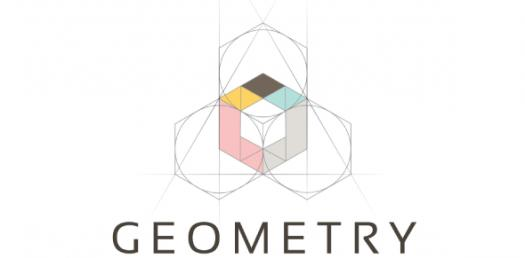# Geometry Trivia: Trivia Test On Shapes And Lines! Quiz

9 Questions | Total Attempts: 167SettingsCreate your own Quiz.

• 1.
Which among the following is not a three-dimensional shape?
• A.

Circle

• B.

Cube

• C.

Cylinder

• D.

Cuboid

• 2.
Select all two dimensional shapes.
• A.

Circle

• B.

Cube

• C.

Pentagon

• D.

Square

• E.

Triangle

• F.

Prism

• G.

Cone

• 3.
A two dimensional shape with six sides is called as
• 4.
A circle is a polygon.
• A.

True

• B.

False

• 5.
A line has two end points.
• A.

True

• B.

False

• 6.
A ray has one end point.
• A.

True

• B.

False

• 7.
_______ is used to represent a point.
• 8.
The basic 2D shape of a cube is
• A.

Triangle

• B.

Square

• C.

Rectangle

• D.

Pentagon

Related TopicsBack to top
×

Wait!
Here's an interesting quiz for you.